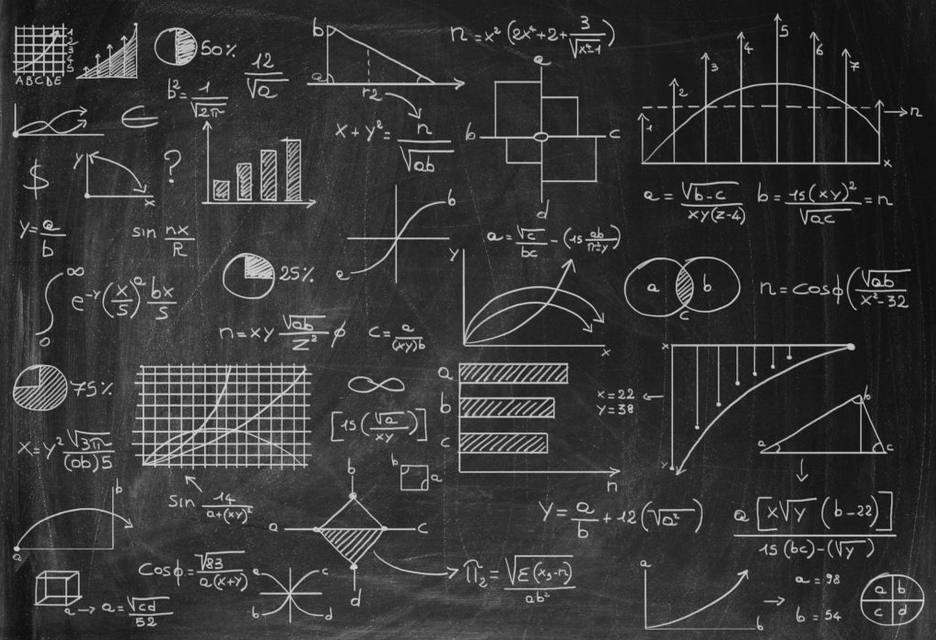10/10/2020

For all the Mathematicians, Physicists, Economists, Data Scientists, Computer Scientists, and every other kind of scientist our there that has worked with math typesetting programs like $\LaTeX$, if you have ever wanted to host your own techy/sciency/mathy blog platform for a very cheap price (pretty much just free), then I am proud to say that the Markdown renderer behind Noiiice has math typesetting capabilities with $\KaTeX$ now!

The Inspiration

Originally, Noiiice's Markdown renderer was using markedjs. Now, marked is a great Markdown renderer, and is pretty popular on GitHub with 23.4k stars at the time of this writing. Marked along with highlightjs allowed for some nice technical writing with code displays on the Noiiice platform. But I felt there were some things missing from the Markdown capabilities here. I couldn't type subscripts or superscripts, and I couldn't make footnotes in the familiar ways that you would find say on GitHub's Markdown rendering.

Being a math geek, I kinda really wanted the ability to typeset at least some simple math stuff. With such interest, I thought this might be a great opportunity to contribute to Noiiice. I really didn't know where to start, so I spent a couple weeks during my freetime looking over math typesetting engines for the browser like MathJax and KaTeX. They each have their pros and cons, and they both seem to be quite mature for my needs. Now the question was how to get one of these to work with markedjs?

Markedjs advertises itself as being light-weight with as few dependencies as possible, and relatively painless to extend it's capabilities. Now, "relatively painless" is indeed the phrase used on their documentation site, but with my Javascript skills, my attempt at extending marked with a math typesetting capability for Noiiice turned out to be quite painful for myself. What were the alternatives?

Markdown-it seemed to be the only other alternative with similar functionality to marked, and perhaps easy enough for me to consider messing around with it. While markdown-it only has about 10.4k Github stars, it showed some promise with it's network of community written plugins available from npm, and it even had one for KaTeX. Voila! With a bit of fidgeting around with Noiiice's Markdown component, I was finally able to get some beautiful math typesetting going by changing the Markdown renderer.

I learned quite a bit, and was very excited that I had done something, in a programming language that I am not quite as comfortable in as I am in others, that could possibly bring value and enhance the capabilities of this cool open source project - Noiiice. Also, a big thanks to the Noiiice Github maintainer, Dylan Allen, for accepting my pull request to make the math typesetting functionality a reality!

Some Examples

We can do some simple inline typesetting by enclosing the math within single tags like, $\frac{1}{2}x^2 - 3x + 1 = 0$ for $x \in \RR$. We can also do some block or display style type setting by enclosing the math inside of double tags like $i \hbar \frac{\partial}{\partial t}\Psi(\mathbf{r},t) = \hat H \Psi(\mathbf{r},t)$ or even a bit more complex and aligned on the "=" signs like \begin{aligned} \nabla \times \vec{\mathbf{B}} -\, \frac1c\, \frac{\partial\vec{\mathbf{E}}}{\partial t} & = \frac{4\pi}{c}\vec{\mathbf{j}} \\[1em] \nabla \cdot \vec{\mathbf{E}} & = 4 \pi \rho \\[0.5em] \nabla \times \vec{\mathbf{E}}\, +\, \frac1c\, \frac{\partial\vec{\mathbf{B}}}{\partial t} & = \vec{\mathbf{0}} \\[1em] \nabla \cdot \vec{\mathbf{B}} & = 0 \end{aligned} The $\LaTeX$ for the expressions above are given in the code block blow. # The inline quadratic equation: \frac{1}{2}x^2 - 3x + 1 = 0 for x \in \RR ## Note the \RR above is actually a LaTeX macro for \mathbb{R} which I had configured in the backend markdown-it-katex Javascript plugin. # The Schrodinger's Equation display: i \hbar \frac{\partial}{\partial t}\Psi(\mathbf{r},t) = \hat H \Psi(\mathbf{r},t) $$# The Maxwell's Equations display:$$ \begin{aligned} \nabla \times \vec{\mathbf{B}} -\, \frac1c\, \frac{\partial\vec{\mathbf{E}}}{\partial t} & = \frac{4\pi}{c}\vec{\mathbf{j}} \\[1em] \nabla \cdot \vec{\mathbf{E}} & = 4 \pi \rho \\[0.5em] \nabla \times \vec{\mathbf{E}}\, +\, \frac1c\, \frac{\partial\vec{\mathbf{B}}}{\partial t} & = \vec{\mathbf{0}} \\[1em] \nabla \cdot \vec{\mathbf{B}} & = 0 \end{aligned}\$

If you're interested in learning more about $\KaTeX$ functionality, then check out some of the documentation.

What's Next?

Well, it's actually been an interesting couple of months since I last wrote a blog post, and now that I can render math on here, I am looking to create a bit of machine learning content coming up. Stay tuned!